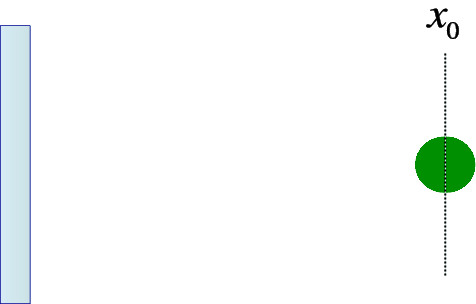## Chapter 7 The Harmonic oscillator

You may be familiar with several examples of harmonic oscillators form classical mechanics, such as particles on a spring or the pendulum for small deviation from equilibrium, etc.Figure 7.1: The mass on the spring and its equilibrium position

Let me look at the characteristics of one such example, a particle of mass $m$ on a spring. When the particle moves a distance $x$ away from the equilibrium position ${x}_{0}$, there will be a restoring force $-kx$ pushing the particle back ( $x>0$ right of equilibrium, and $x<0$ on the left). This can be derived from a potential

 $V\left(x\right)=\frac{1}{2}k{x}^{2}.$ (7.1)

Actually we shall write $k=m{\omega }^{2}$. The equation of motion

 $mẍ=-m{\omega }^{2}x$ (7.2)

has the solution

 $x\left(t\right)=Acos\left(\omega t\right)+Bsin\left(\omega t\right).$ (7.3)

We now consider how this system behaves quantum-mechanically.